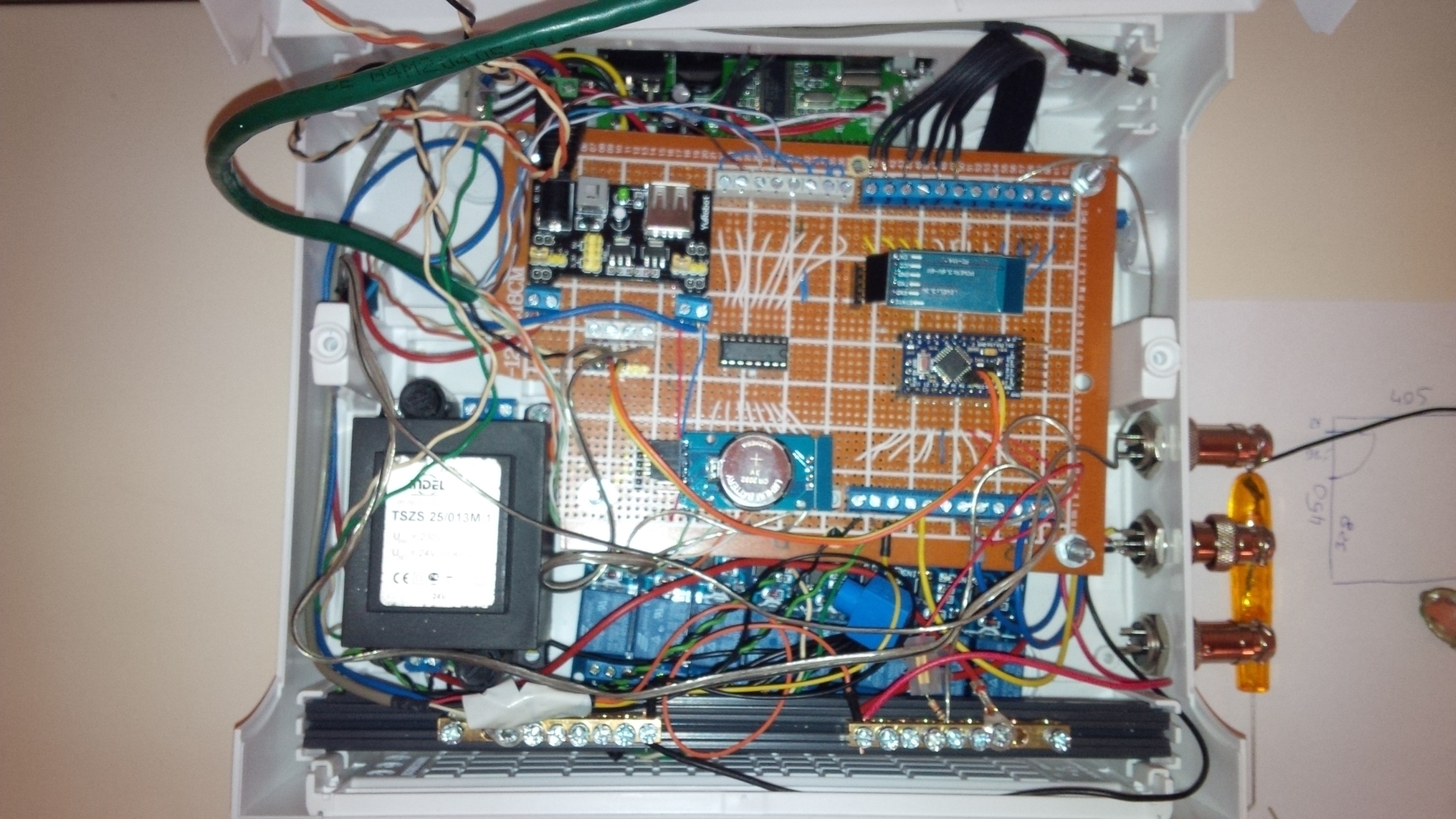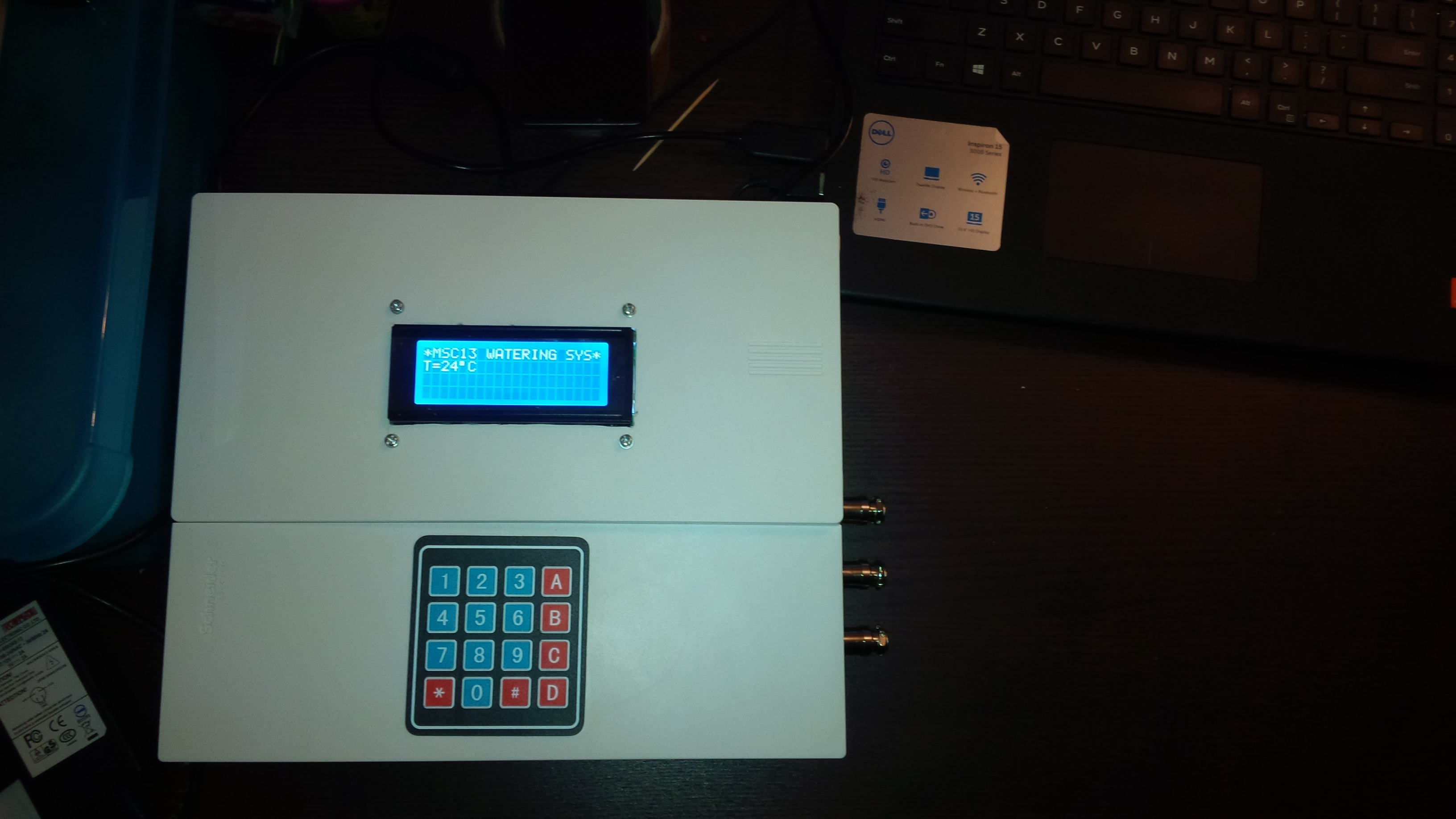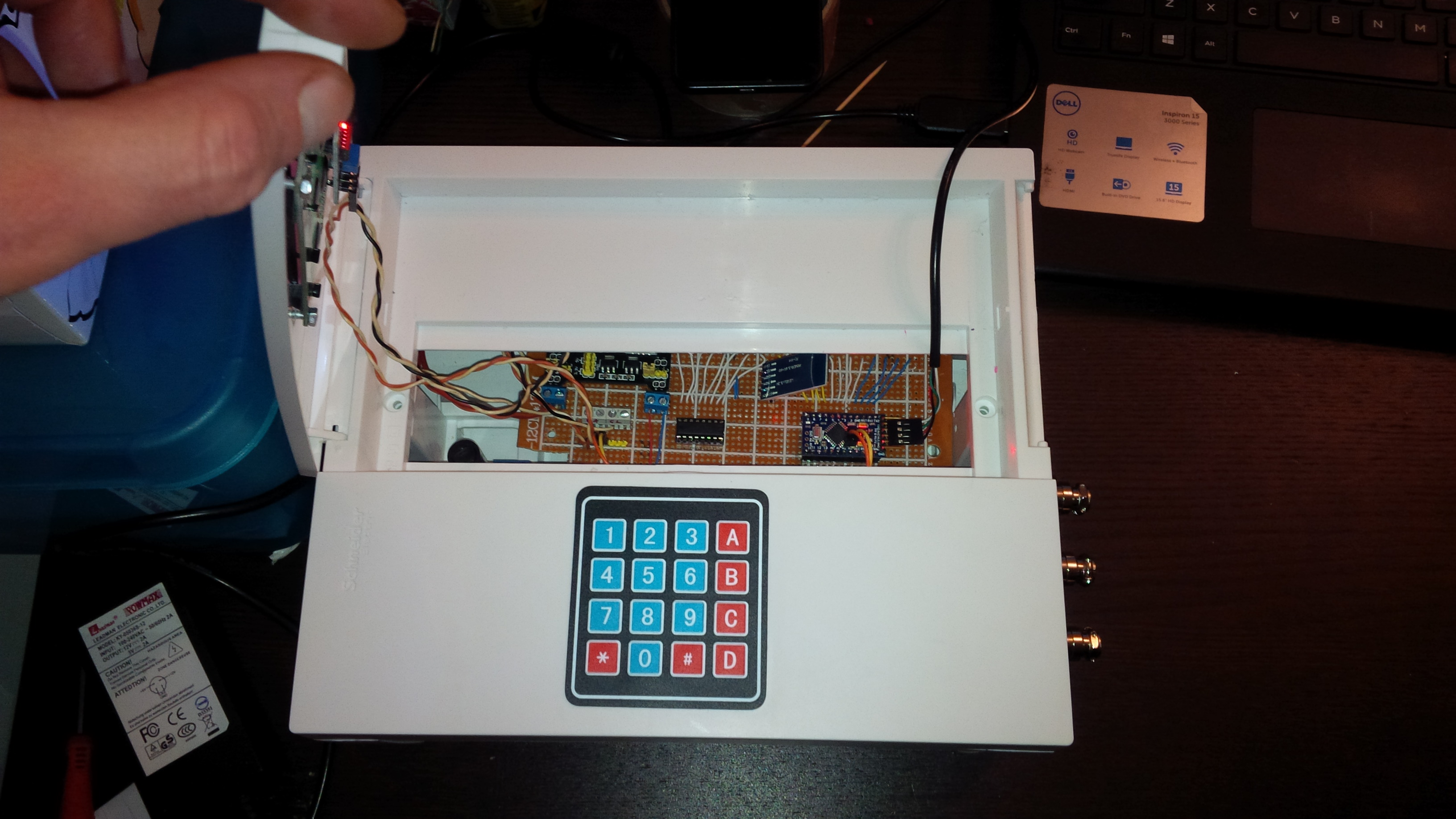# BMP280 + I2C

• Hello,
This is my first post here, so please "be nice" to me.
So far I built several nodes for temperature measurement, LED control, RF433 to control my outside sockets, in future my heating system will be based on reading from temperature nodes. Finishing at the moment irrigation controller for 8 zones ( think to post it when finish).

Few days ago I received my BMP280 sensor
for my outdoor weather station but couldn't find any projects here so I made it by myself (partly).
So if anyone of you is going to use this sensor you may use this.
Tested on Uno and works. I use Domoticz and it works just fine.

``````//                  BMP280 I2C
// this program is for BMP280 library :

/*
Bosch BMP280 pressure sensor library for the Arduino microcontroller.
This library uses I2C connection.

Uses floating-point equations from BMP280 datasheet.

modified by mhafuzul islam

version 1.01		 16/9/2014 initial version
https://github.com/mahfuz195/BMP280-Arduino-Library/tree/master/BMP280

Our example code uses the "pizza-eating" license. You can do anything
you like with this code. No really, anything. If you find it useful,
buy me italian pizza someday.

I also used some parts from oryginal MySensors PressureSensor for BMP085 module

To use with MySensors 2.0.0 dev.branch

* REVISION HISTORY
* Version 1.0 - Henrik Ekblad
* Version 1.1 - Marek Dajnowicz

*/

// Enable debug prints to serial monitor
#define MY_DEBUG
//#define MY_REPEATER_FEATURE

// Enable and select radio type attached

//#define MY_NODE_ID 33

//#define MY_PARENT_NODE_ID

#include <SPI.h>
#include <MySensor.h>
#include "BMP280.h"
#include "Wire.h"

#define P0 1010.6 // sea level pressure for my place
#define CHILD_ID_TEMP 1
#define CHILD_ID_PRESS 2

const char *weather[] = { "stable", "sunny", "cloudy", "unstable", "thunderstorm", "unknown" };
enum FORECAST
{
STABLE = 0,			// "Stable Weather Pattern"
SUNNY = 1,			// "Slowly rising Good Weather", "Clear/Sunny "
CLOUDY = 2,			// "Slowly falling L-Pressure ", "Cloudy/Rain "
UNSTABLE = 3,		// "Quickly rising H-Press",     "Not Stable"
THUNDERSTORM = 4,	// "Quickly falling L-Press",    "Thunderstorm"
UNKNOWN = 5			// "Unknown (More Time needed)
};

BMP280 bmp; // call for sensor
// for forecast
float lastPressure = -1;
float lastTemp = -1;
int lastForecast = -1;
const int LAST_SAMPLES_COUNT = 5;
float lastPressureSamples[LAST_SAMPLES_COUNT];
// this CONVERSION_FACTOR is used to convert from Pa to kPa in forecast algorithm
// get kPa/h be dividing hPa by 10
#define CONVERSION_FACTOR (1.0/10.0)
int minuteCount = 0;
bool firstRound = true;
// average value is used in forecast algorithm.
float pressureAvg;
// average after 2 hours is used as reference value for the next iteration.
float pressureAvg2;
float dP_dt;

// MyMessage to controler
MyMessage msgT1(CHILD_ID_TEMP, V_TEMP);
MyMessage msgP1(CHILD_ID_PRESS, V_PRESSURE);
MyMessage msgF1(CHILD_ID_PRESS, V_FORECAST);

// values for ServerUpdate, unmark when not using smartSleep
//const unsigned long tUpdate = 60000; // update interval
//unsigned long t0;

void presentation()
{
// Send the sketch version information to the gateway and Controller
sendSketchInfo("OUTSIDE P&T", "1.1");
present(CHILD_ID_TEMP, S_TEMP);
present(CHILD_ID_PRESS, S_BARO);
}

void setup()
{
delay(500);// just in case
if (!bmp.begin())
{
Serial.println("BMP init failed!");
while (1);
}
else Serial.println("BMP init success!");
bmp.setOversampling(4);
ServerUpdate(); // for first data reading and sending to controler
}

void loop()
{ smartSleep(60000); // adjust sleeping time here 1 minute in this case
ServerUpdate();
//  if ((millis() - t0) > tUpdate) ServerUpdate();
}

void ServerUpdate() // used to read sensor data and send it to controler
{
double T, P;
char result = bmp.startMeasurment();

if (result != 0) {
delay(result);
result = bmp.getTemperatureAndPressure(T, P);

int forecast = sample(P);
if (result != 0)
{
// double A = bmp.altitude(P, P0); // this is to get barometric Altitude, not used in this program
send(msgT1.set(T, 1));
send(msgP1.set(P, 1));
send(msgF1.set(weather[forecast]));

// unmark for debuging
//   Serial.print("T = \t"); Serial.print(T, 1); Serial.print(" degC\t");
//   Serial.print("P = \t"); Serial.print(P, 1); Serial.print(" mBar\t");
//   Serial.print("A = \t"); Serial.print(A, 1); Serial.println(" m");
//   Serial.print("F = \t"); Serial.print(weather[forecast]); Serial.println(" ?");
}
else {
Serial.println("Error.");
}
}
else {
Serial.println("Error.");
}

// t0 = millis(); used without smartSleep
}

float getLastPressureSamplesAverage()
{
float lastPressureSamplesAverage = 0;
for (int i = 0; i < LAST_SAMPLES_COUNT; i++)
{
lastPressureSamplesAverage += lastPressureSamples[i];
}
lastPressureSamplesAverage /= LAST_SAMPLES_COUNT;

return lastPressureSamplesAverage;
}

// Algorithm found here
// http://www.freescale.com/files/sensors/doc/app_note/AN3914.pdf
// Pressure in hPa -->  forecast done by calculating kPa/h
int sample(float pressure)
{
// Calculate the average of the last n minutes.
int index = minuteCount % LAST_SAMPLES_COUNT;
lastPressureSamples[index] = pressure;

minuteCount++;
if (minuteCount > 185)
{
minuteCount = 6;
}

if (minuteCount == 5)
{
pressureAvg = getLastPressureSamplesAverage();
}
else if (minuteCount == 35)
{
float lastPressureAvg = getLastPressureSamplesAverage();
float change = (lastPressureAvg - pressureAvg) * CONVERSION_FACTOR;
if (firstRound) // first time initial 3 hour
{
dP_dt = change * 2; // note this is for t = 0.5hour
}
else
{
dP_dt = change / 1.5; // divide by 1.5 as this is the difference in time from 0 value.
}
}
else if (minuteCount == 65)
{
float lastPressureAvg = getLastPressureSamplesAverage();
float change = (lastPressureAvg - pressureAvg) * CONVERSION_FACTOR;
if (firstRound) //first time initial 3 hour
{
dP_dt = change; //note this is for t = 1 hour
}
else
{
dP_dt = change / 2; //divide by 2 as this is the difference in time from 0 value
}
}
else if (minuteCount == 95)
{
float lastPressureAvg = getLastPressureSamplesAverage();
float change = (lastPressureAvg - pressureAvg) * CONVERSION_FACTOR;
if (firstRound) // first time initial 3 hour
{
dP_dt = change / 1.5; // note this is for t = 1.5 hour
}
else
{
dP_dt = change / 2.5; // divide by 2.5 as this is the difference in time from 0 value
}
}
else if (minuteCount == 125)
{
float lastPressureAvg = getLastPressureSamplesAverage();
pressureAvg2 = lastPressureAvg; // store for later use.
float change = (lastPressureAvg - pressureAvg) * CONVERSION_FACTOR;
if (firstRound) // first time initial 3 hour
{
dP_dt = change / 2; // note this is for t = 2 hour
}
else
{
dP_dt = change / 3; // divide by 3 as this is the difference in time from 0 value
}
}
else if (minuteCount == 155)
{
float lastPressureAvg = getLastPressureSamplesAverage();
float change = (lastPressureAvg - pressureAvg) * CONVERSION_FACTOR;
if (firstRound) // first time initial 3 hour
{
dP_dt = change / 2.5; // note this is for t = 2.5 hour
}
else
{
dP_dt = change / 3.5; // divide by 3.5 as this is the difference in time from 0 value
}
}
else if (minuteCount == 185)
{
float lastPressureAvg = getLastPressureSamplesAverage();
float change = (lastPressureAvg - pressureAvg) * CONVERSION_FACTOR;
if (firstRound) // first time initial 3 hour
{
dP_dt = change / 3; // note this is for t = 3 hour
}
else
{
dP_dt = change / 4; // divide by 4 as this is the difference in time from 0 value
}
pressureAvg = pressureAvg2; // Equating the pressure at 0 to the pressure at 2 hour after 3 hours have past.
firstRound = false; // flag to let you know that this is on the past 3 hour mark. Initialized to 0 outside main loop.
}

int forecast = UNKNOWN;
if (minuteCount < 35 && firstRound) //if time is less than 35 min on the first 3 hour interval.
{
forecast = UNKNOWN;
}
else if (dP_dt < (-0.25))
{
forecast = THUNDERSTORM;
}
else if (dP_dt > 0.25)
{
forecast = UNSTABLE;
}
else if ((dP_dt > (-0.25)) && (dP_dt < (-0.05)))
{
forecast = CLOUDY;
}
else if ((dP_dt > 0.05) && (dP_dt < 0.25))
{
forecast = SUNNY;
}
else if ((dP_dt > (-0.05)) && (dP_dt < 0.05))
{
forecast = STABLE;
}
else
{
forecast = UNKNOWN;
}

// uncomment when debugging
//Serial.print(F("Forecast at minute "));
//Serial.print(minuteCount);
//Serial.print(F(" dP/dt = "));
//Serial.print(dP_dt);
//Serial.print(F("kPa/h --> "));
//Serial.println(weather[forecast]);

return forecast;
}
``````

###Breakout Temperature Humidity Barometric Pressure BME280 Digital Sensor Module

\$3.78
7007 available

• Are you using a BMP280 (temp + pressure) or as you linked a BME280 (temp + hum + pressure)?

If you have a BME280 have a look at http://forum.mysensors.org/topic/2821/myweathergatewayesp8266-1-6-dev-branch for adding the humidity readings.

• @marekd Welcome on the forum. And thank you for sharing your sketch. Would love to see some pictures of your irrigation controller.

• @emc2 , must check it. the link is what I purchased.

• @TheoL, I have some pictures of my Ver 1.0 (before I found MySensors), but anyway it was based on standard Promini + RTC, Bluetooth, LCD 16x2, Keyboard and some other sensors.
And it was a bit messy with all the wires.

Now is going to be based on Mega256, NRF24 ... still under development.• @emc2, Thank you for your comment, my sensor is BME280 so now I can use hum as well.
I made quick upgrade of my sketch. Had to modify I2C address (good that you wrote that in your post), I change in the library for 0x76 instead.
Here is my sketch for BME280:

``````/*                  BME280 I2C for MySensors

// this program is for BMEP280 library :Adafriut BME280

I use some parts from oryginal MySensors PressureSensor for BMP085 module

To use with MySensors 2.0.0 dev.branch

* REVISION HISTORY
* Version 1.0 - Henrik Ekblad
* Version 1.2 - Marek Dajnowicz

*/

// Enable debug prints to serial monitor
#define MY_DEBUG
//#define MY_REPEATER_FEATURE

// Enable and select radio type attached

//#define MY_NODE_ID 33

//#define MY_PARENT_NODE_ID

#include <SPI.h>
#include <MySensor.h>
#include <Adafruit_BME280.h> // I had to change I2C address in library for 0x76 (line 32)
#include "Wire.h"

Adafruit_BME280 bme; // I2C

#define CHILD_ID_HUM 0
#define CHILD_ID_TEMP 1
#define CHILD_ID_PRESS 2

const char *weather[] = { "stable", "sunny", "cloudy", "unstable", "thunderstorm", "unknown" };
enum FORECAST
{
STABLE = 0,			// "Stable Weather Pattern"
SUNNY = 1,			// "Slowly rising Good Weather", "Clear/Sunny "
CLOUDY = 2,			// "Slowly falling L-Pressure ", "Cloudy/Rain "
UNSTABLE = 3,		// "Quickly rising H-Press",     "Not Stable"
THUNDERSTORM = 4,	// "Quickly falling L-Press",    "Thunderstorm"
UNKNOWN = 5			// "Unknown (More Time needed)
};

// for forecast - untouched by me :)

float lastPressure = -1;
float lastTemp = -1;
int lastForecast = -1;
const int LAST_SAMPLES_COUNT = 5;
float lastPressureSamples[LAST_SAMPLES_COUNT];
// this CONVERSION_FACTOR is used to convert from Pa to kPa in forecast algorithm
// get kPa/h be dividing hPa by 10
#define CONVERSION_FACTOR (1.0/10.0)
int minuteCount = 0;
bool firstRound = true;
// average value is used in forecast algorithm.
float pressureAvg;
// average after 2 hours is used as reference value for the next iteration.
float pressureAvg2;
float dP_dt;

// MyMessage to controler
MyMessage msgT1(CHILD_ID_TEMP, V_TEMP);
MyMessage msgP1(CHILD_ID_PRESS, V_PRESSURE);
MyMessage msgF1(CHILD_ID_PRESS, V_FORECAST);
MyMessage msgH1(CHILD_ID_HUM, V_HUM);

void presentation()
{
// Send the sketch version information to the gateway and Controller
sendSketchInfo("OUTSIDE P&T&H", "1.2");
present(CHILD_ID_TEMP, S_TEMP);
present(CHILD_ID_PRESS, S_BARO);
present(CHILD_ID_HUM, S_HUM);
}

void setup()
{
delay(500);// just in case
if (!bme.begin())
{
Serial.println("BME init failed!");
while (1);
}
else Serial.println("BME init success!");

ServerUpdate(); // for first data reading and sending to controler
}

void loop()
{
smartSleep(60000); // adjust sleeping time here 1 minute in this case
ServerUpdate();
}

void ServerUpdate() // used to read sensor data and send it to controller
{
double T, P, H;

int forecast = sample(P);
send(msgT1.set(T, 1));
send(msgP1.set(P, 1));
send(msgH1.set(H,1));
send(msgF1.set(weather[forecast]));

// unmark for debuging
Serial.print("T = \t"); Serial.print(T, 1); Serial.print(" degC\t");
Serial.print("P = \t"); Serial.print(P, 1); Serial.print(" mBar\t");
Serial.print("F = \t"); Serial.print(weather[forecast]); Serial.println(" ?");
}

float getLastPressureSamplesAverage()
{
float lastPressureSamplesAverage = 0;
for (int i = 0; i < LAST_SAMPLES_COUNT; i++)
{
lastPressureSamplesAverage += lastPressureSamples[i];
}
lastPressureSamplesAverage /= LAST_SAMPLES_COUNT;

return lastPressureSamplesAverage;
}

// Algorithm found here
// http://www.freescale.com/files/sensors/doc/app_note/AN3914.pdf
// Pressure in hPa -->  forecast done by calculating kPa/h
int sample(float pressure)
{
// Calculate the average of the last n minutes.
int index = minuteCount % LAST_SAMPLES_COUNT;
lastPressureSamples[index] = pressure;

minuteCount++;
if (minuteCount > 185)
{
minuteCount = 6;
}

if (minuteCount == 5)
{
pressureAvg = getLastPressureSamplesAverage();
}
else if (minuteCount == 35)
{
float lastPressureAvg = getLastPressureSamplesAverage();
float change = (lastPressureAvg - pressureAvg) * CONVERSION_FACTOR;
if (firstRound) // first time initial 3 hour
{
dP_dt = change * 2; // note this is for t = 0.5hour
}
else
{
dP_dt = change / 1.5; // divide by 1.5 as this is the difference in time from 0 value.
}
}
else if (minuteCount == 65)
{
float lastPressureAvg = getLastPressureSamplesAverage();
float change = (lastPressureAvg - pressureAvg) * CONVERSION_FACTOR;
if (firstRound) //first time initial 3 hour
{
dP_dt = change; //note this is for t = 1 hour
}
else
{
dP_dt = change / 2; //divide by 2 as this is the difference in time from 0 value
}
}
else if (minuteCount == 95)
{
float lastPressureAvg = getLastPressureSamplesAverage();
float change = (lastPressureAvg - pressureAvg) * CONVERSION_FACTOR;
if (firstRound) // first time initial 3 hour
{
dP_dt = change / 1.5; // note this is for t = 1.5 hour
}
else
{
dP_dt = change / 2.5; // divide by 2.5 as this is the difference in time from 0 value
}
}
else if (minuteCount == 125)
{
float lastPressureAvg = getLastPressureSamplesAverage();
pressureAvg2 = lastPressureAvg; // store for later use.
float change = (lastPressureAvg - pressureAvg) * CONVERSION_FACTOR;
if (firstRound) // first time initial 3 hour
{
dP_dt = change / 2; // note this is for t = 2 hour
}
else
{
dP_dt = change / 3; // divide by 3 as this is the difference in time from 0 value
}
}
else if (minuteCount == 155)
{
float lastPressureAvg = getLastPressureSamplesAverage();
float change = (lastPressureAvg - pressureAvg) * CONVERSION_FACTOR;
if (firstRound) // first time initial 3 hour
{
dP_dt = change / 2.5; // note this is for t = 2.5 hour
}
else
{
dP_dt = change / 3.5; // divide by 3.5 as this is the difference in time from 0 value
}
}
else if (minuteCount == 185)
{
float lastPressureAvg = getLastPressureSamplesAverage();
float change = (lastPressureAvg - pressureAvg) * CONVERSION_FACTOR;
if (firstRound) // first time initial 3 hour
{
dP_dt = change / 3; // note this is for t = 3 hour
}
else
{
dP_dt = change / 4; // divide by 4 as this is the difference in time from 0 value
}
pressureAvg = pressureAvg2; // Equating the pressure at 0 to the pressure at 2 hour after 3 hours have past.
firstRound = false; // flag to let you know that this is on the past 3 hour mark. Initialized to 0 outside main loop.
}

int forecast = UNKNOWN;
if (minuteCount < 35 && firstRound) //if time is less than 35 min on the first 3 hour interval.
{
forecast = UNKNOWN;
}
else if (dP_dt < (-0.25))
{
forecast = THUNDERSTORM;
}
else if (dP_dt > 0.25)
{
forecast = UNSTABLE;
}
else if ((dP_dt > (-0.25)) && (dP_dt < (-0.05)))
{
forecast = CLOUDY;
}
else if ((dP_dt > 0.05) && (dP_dt < 0.25))
{
forecast = SUNNY;
}
else if ((dP_dt > (-0.05)) && (dP_dt < 0.05))
{
forecast = STABLE;
}
else
{
forecast = UNKNOWN;
}

// uncomment when debugging
//Serial.print(F("Forecast at minute "));
//Serial.print(minuteCount);
//Serial.print(F(" dP/dt = "));
//Serial.print(dP_dt);
//Serial.print(F("kPa/h --> "));
//Serial.println(weather[forecast]);

return forecast;
}
``````

• @marekd It looks great. Nice job.

• @marekd glad it was useful.

You may not need to change the address in the library.
If you add these 2 lines after #include <Adafruit_BME280.h> it should work without modifications of the library and so survive any future library updates.

``````#undef BME280_ADDRESS         // Undef BME280_ADDRESS from the BME280 library to easily override I2C address
#define BME280_ADDRESS (0x76) // Low = 0x76 , High = 0x77 (default on adafruit and sparkfun BME280 modules, default for library)
``````

• @marekd

Your irrigation controller looks really neat! I like that box man! Where did you get it?

• @TRS-80 Thanks. The box is MINI PRAGMA MIP12112 SCHNEIDER ELECTRIC got it from LeroyMerlin

• @emc2 said in BMP280 + I2C:

@marekd glad it was useful.

You may not need to change the address in the library.
If you add these 2 lines after #include <Adafruit_BME280.h> it should work without modifications of the library and so survive any future library updates.

``````#undef BME280_ADDRESS         // Undef BME280_ADDRESS from the BME280 library to easily override I2C address
#define BME280_ADDRESS (0x76) // Low = 0x76 , High = 0x77 (default on adafruit and sparkfun BME280 modules, default for library)
``````

Thanks for this good idea, but it doesn't always work.
It's better to add the define before the include, in the sketch.
And to replace in the lib
by
#endif
Of course if library updates...